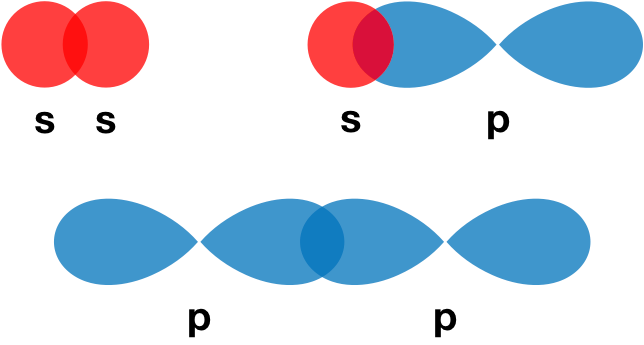# Problem: Label the bonds in XeF4 using the σ sigmaand π pinotation followed by the type of overlapping orbitals.

###### FREE Expert Solution

We’re being asked to label the bonds in XeF4 using the σ and π notation followed by the type of overlapping orbitals.

Recall that σ (sigma) bonds are the single bonds in a molecule. They are formed from the end-on overlap of orbitals.For this problem, we need to do the following steps:

Step 1: Determine the central atom in the molecule.

Step 2: Calculate the total number of valence electrons present.

Step 3: Draw the Lewis structure for the molecule.

Step 4: Determine the hybridization of the central atom.

Step 5: Determine the orbitals that overlap to form the sigma bonds.

82% (311 ratings)###### Problem Details

Label the bonds in XeF4 using the σ and π notation followed by the type of overlapping orbitals.

Frequently Asked Questions

What scientific concept do you need to know in order to solve this problem?

Our tutors have indicated that to solve this problem you will need to apply the Valence Shell Electron Pair Repulsion Theory concept. You can view video lessons to learn Valence Shell Electron Pair Repulsion Theory. Or if you need more Valence Shell Electron Pair Repulsion Theory practice, you can also practice Valence Shell Electron Pair Repulsion Theory practice problems.

What professor is this problem relevant for?

Based on our data, we think this problem is relevant for Professor Ray's class at UIUC.